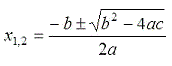We are going to create now a Matlab program that calculates the quadratic roots (roots of quadratic equations). The equation must be in the following form:

ax2 + bx + c = 0

where a, b, and c are real coefficients.

The formula used to calculate the roots is:Naturally, we have to deliver two x-values.

The following video shows you an example of what we want to accomplish. We use an online calculator to solve this problem. After the video, we show you the equivalent code that we have to implement to get the same results.

The m-file that we might use to accomplish this task is very simple:

function x = rqe(a,b,c)
x(1) = (-b + sqrt(b^2 - 4 * a * c))/(2*a);
x(2) = (-b - sqrt(b^2 - 4 * a * c))/(2*a);

We enter the coefficients as parameters when we call the function. We assign to variables x(1) and x(2) the calculated values using the formula, and the returning result x is a vector containing x(1) and x(2).

We could use the following Matlab code instead, to return two separate variables:

function [x,y] = rqe2(a,b,c)
x = (-b + sqrt(b^2 - 4 * a * c))/(2*a);
y = (-b - sqrt(b^2 - 4 * a * c))/(2*a);

If we want to compute the roots of the following expression:

2x2 + x - 1 = 0

We can call our function (first code) like this:

x = rqe(2, 1, -1)

and we get from Matlab:

x =     0.5000   -1.0000

We can call our second function (second code above) like this:

[m, n] = rqe2(2, 1, -1)

and we get from Matlab:

m =     0.5000

n =     -1